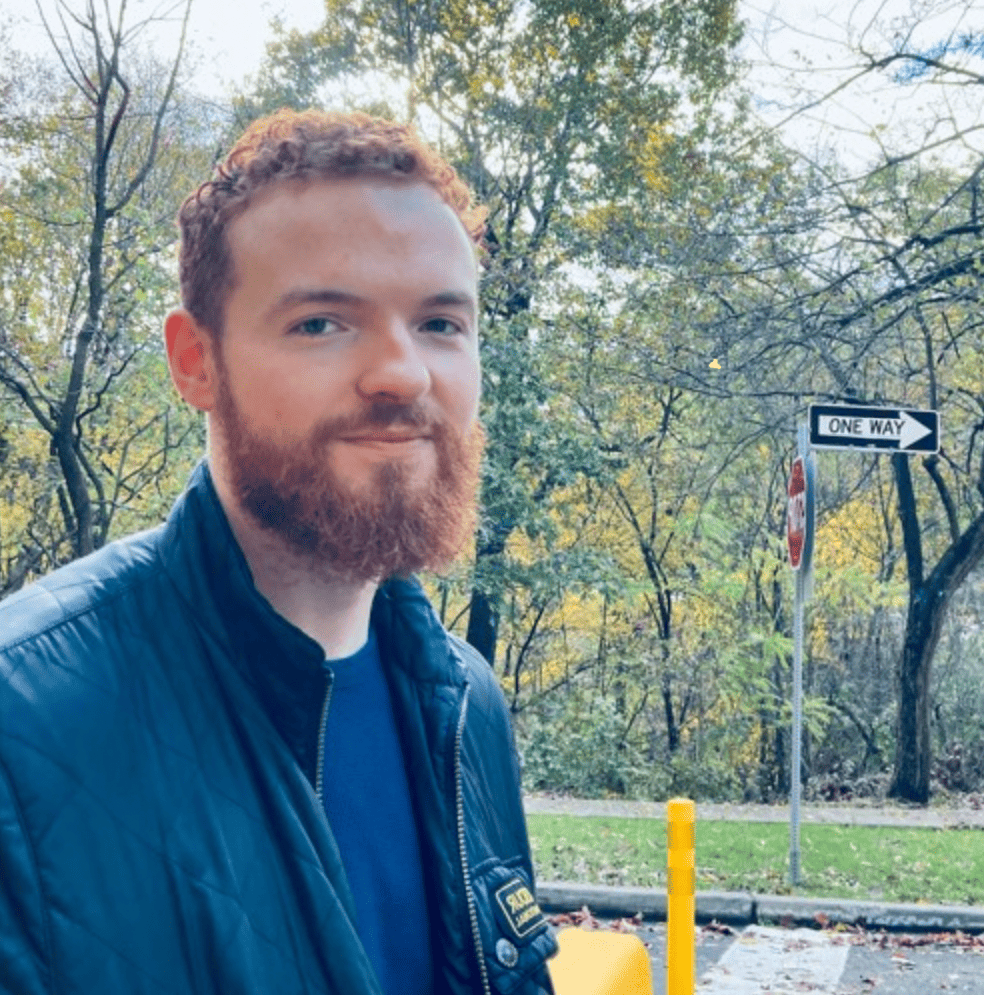Timezone: »

Poster
Weighted Tallying Bandits: Overcoming Intractability via Repeated Exposure Optimality
Dhruv Malik · Conor Igoe · Yuanzhi Li · Aarti Singh

Thu Jul 27 04:30 PM -- 06:00 PM (PDT) @ Exhibit Hall 1 #328
In human-interactive applications of online learning, a human's preferences or abilities are often a function of the algorithm's recent actions. Motivated by this, a significant line of work has formalized settings where an action's loss is a function of the number of times it was played in the prior $m$ timesteps, where $m$ corresponds to a bound on human memory capacity. To more faithfully capture decay of human memory with time, we introduce the Weighted Tallying Bandit (WTB), which generalizes this setting by requiring that an action's loss is a function of a *weighted* summation of the number of times it was played in the last $m$ timesteps. WTB is intractable without further assumption. So we study it under Repeated Exposure Optimality (REO), a condition requiring the existence of an action that when repetitively played will eventually yield smaller loss than any other action sequence. We study the minimization of complete policy regret (CPR), which is the strongest notion of regret, in WTB under REO. Since $m$ is often unknown, we only assume access to an upper bound $M$ on $m$. We show that for problems with $K$ actions and horizon $T$, a simple modification of the successive elimination algorithm has $\mathcal{O} \left( \sqrt{KT} + (m+M)K \right)$ CPR. Upto an additive (in lieu of mutliplicative) factor in $(m+M)K$, this recovers the classical guarantee for the far simpler stochastic multi-armed bandit with traditional regret. We additionally show that in our setting, any algorithm will suffer additive CPR of $\Omega \left( mK + M \right)$, demonstrating our result is near optimal. Our method is computationally efficient, and we experimentally demonstrate its practicality and superiority over various baselines.

#### Author Information

##### Conor Igoe (CMU Machine Learning Department)I work primarily on Reinforcement Learning and Design of Experiments, although I am broadly interested in Uncertainty in Machine Learning.